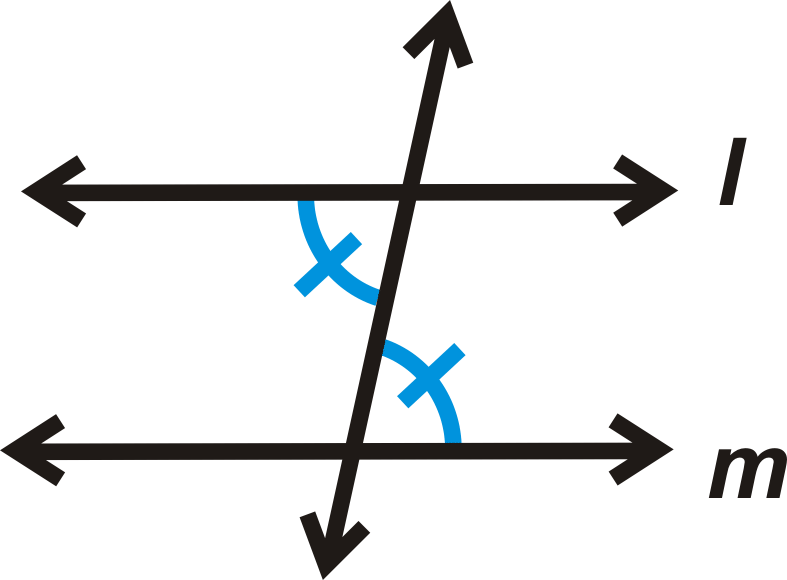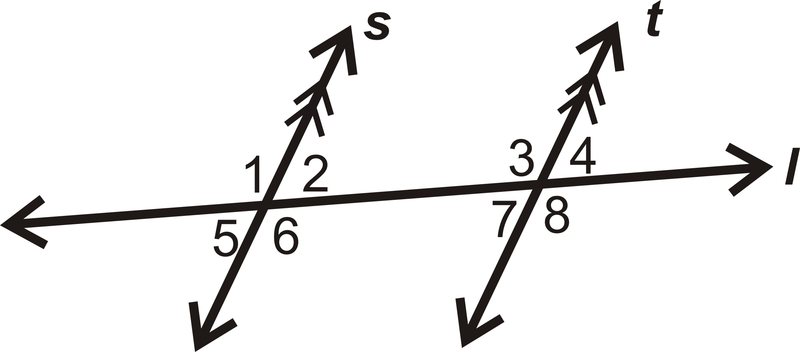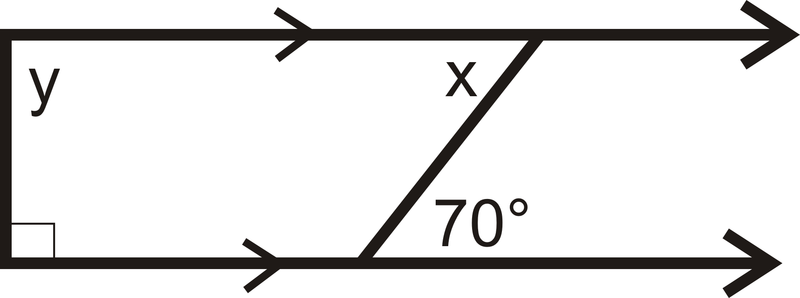# 3.5: Alternate Interior Angles

•• Contributed by CK12
• CK12

Angles on opposite sides of a transversal, but inside the lines it intersects.

Alternate interior angles are two angles that are on the interior of $$l$$ and $$m$$, but on opposite sides of the transversal.Figure $$\PageIndex{1}$$

Alternate Interior Angles Theorem: If two parallel lines are cut by a transversal, then the alternate interior angles are congruent.Figure $$\PageIndex{2}$$

If $$l\parallel m$$, then $$\angle 1\cong \angle 2$$

Converse of Alternate Interior Angles Theorem: If two lines are cut by a transversal and the alternate interior angles are congruent, then the lines are parallel.

IfFigure $$\PageIndex{3}$$

then $$l\parallel m$$.

What if you were presented with two angles that are on the interior of two parallel lines cut by a transversal but on opposite sides of the transversal? How would you describe these angles and what could you conclude about their measures?

For Examples $$\PageIndex{1}$$ and $$\PageIndex{2}$$, use the given information to determine which lines are parallel. If there are none, write none. Consider each question individually.Figure $$\PageIndex{4}$$

Example $$\PageIndex{1}$$

$$\angle EAF\cong \angle FJI$$

Solution

None

Example $$\PageIndex{2}$$

$$\angle EFJ\cong \angle FJK$$

Solution

$$\overleftarrow{CG} \parallel \overleftarrow{HK}$$

Example $$\PageIndex{3}$$

Find the value of $$x$$.Figure $$\PageIndex{5}$$

Solution

The two given angles are alternate interior angles and equal.

\begin{align*} (4x−10)^{\circ} &=58^{\circ}\\ 4x &=68 \\ x &=17 \end{align*}

Example $$\PageIndex{4}$$

True or false: alternate interior angles are always congruent.

Solution

This statement is false, but is a common misconception. Remember that alternate interior angles are only congruent when the lines are parallel.

Example $$\PageIndex{5}$$

The angles are alternate interior angles, and must be equal for $$a\parallel b$$. Set the expressions equal to each other and solve.Figure $$\PageIndex{6}$$

Solution

\begin{align*} 3x+16^{\circ} &=5x−54^{\circ} \\ 70&=2x \\ 35 &=x\end{align*}

To make $$a\parallel b$$, $$x=35$$.

### Review

1. Is the angle pair $$\angle 6$$ and $$\angle 3$$ congruent, supplementary or neither?Figure $$\PageIndex{7}$$
2. Give two examples of alternate interior angles in the diagram:Figure $$\PageIndex{8}$$

For 3-4, find the values of $$x$$.

1.Figure $$\PageIndex{9}$$
2.Figure $$\PageIndex{10}$$

For question 5, use the picture below. Find the value of $$x$$.Figure $$\PageIndex{11}$$
1. $$m \angle 4=(5x−33)^{\circ}$$, $$m \angle 5=(2x+60)^{\circ}$$
1. Are lines $$l$$ and $$m$$ parallel? If yes, how do you know?Figure $$\PageIndex{12}$$

For 7-10, what does the value of $$x$$ have to be to make the lines parallel?Figure $$\PageIndex{13}$$
1. $$m \angle 4=(3x−7)^{\circ}$$ and $$m \angle 5=(5x−21)^{\circ}$$
2. $$m \angle 3=(2x−1)^{\circ}$$ and $$m \angle 6=(4x−11)^{\circ}$$
3. $$m \angle 3=(5x−2)^{\circ}$$ and $$m \angle 6=(3x)^{\circ}$$
4. $$m \angle 4=(x−7)^{\circ}$$ and $$m \angle 5=(5x−31)^{\circ}$$

## Vocabulary

Term Definition
alternate interior angles Alternate interior angles are two angles that are on the interior of two different lines, but on the opposite sides of the transversal.
alternate exterior angles Alternate exterior angles are two angles that are on the exterior of two different lines, but on the opposite sides of the transversal.